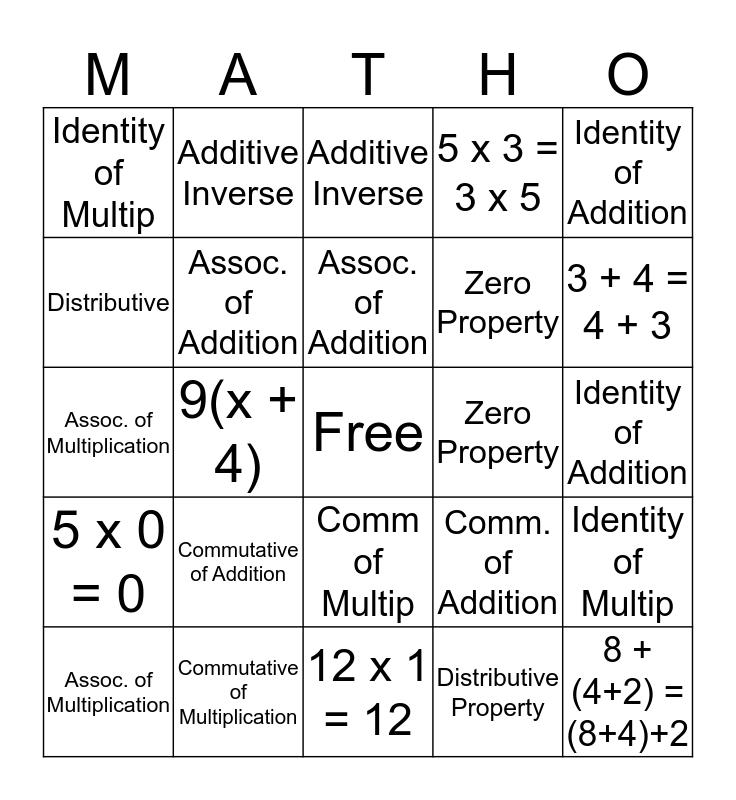# PropertiesThis bingo card has a free space and 24 words: Commutative of Addition, Commutative of Multiplication, Assoc. of Addition, Assoc. of Multiplication, Identity of Addition, Identity of Multip, Distributive, Additive Inverse, Zero Property, Comm of Multip, Zero Property, Assoc. of Multiplication, Comm. of Addition, Identity of Multip, Assoc. of Addition, Distributive Property, Identity of Addition, Additive Inverse, 3 + 4 = 4 + 3, 9(x + 4), 8 +(4+2) = (8+4)+2, 5 x 0 = 0, 5 x 3 = 3 x 5 and 12 x 1 = 12.

⚠ This card has duplicate items: Assoc. of Addition (2), Assoc. of Multiplication (2), Identity of Addition (2), Identity of Multip (2), Additive Inverse (2), Zero Property (2)

## Play Online